﻿ 基于压缩量偏差约束的整体叶盘砂布轮数控抛光路径规划Download PDF文章快速检索 高级检索

A polishing path planning method for blisk with abrasive cloth wheel based on compression deviation constraint
WANG Zhiwei, LIN Xiaojun, SHI Yaoyao, GAO Yuan, ZHANG Yun
School of Mechanical Engineering, Northwestern Polytechnical University, Xi'an 710072, China
Abstract: CNC polishing is the key technology to realize the automation and high efficiency of the surface finishing of the blisk. And the polishing path planning has a significant influence on the surface quality. When polishing the free-form surface, the deviation of radial compression quantity along the contact curve of abrasive cloth wheel leads to poor consistency of material removal quantity. Based on the geometric analysis of the extrusion deformation of the abrasive cloth wheel, a compression deformation distribution model along the contact curve on the free-form surface was established. The optimization model of tool orientation at one cutter location was established, and the improved Partical Swarm Optimization (PSO) was used to solve the problem. The objective function of optimization was evaluated by minimizing the maximum compression deviation along the contact curve. Then the curves of adjacent cutter contact trajectories are calculated. Finally, the correctness and the effectiveness of the proposed method were verified by experiments. The results show that the contour and roughness of the polished area are improved significantly.
Keywords: blisk    free-form surface    NC Polishing    path planning    tool orientation

1 数控抛光路径规划 1.1 砂布轮抛光模型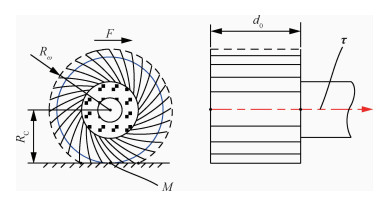图 1 砂布轮模型 Fig. 1 Model of abrasive cloth wheel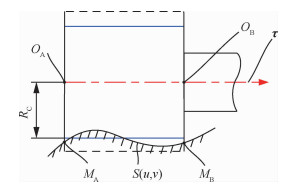图 2 砂布轮抛光曲面 Fig. 2 Polishing surface with abrasive cloth wheel
1.2 曲面参数化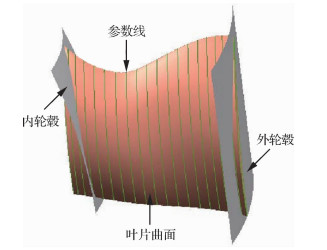图 3 裁剪后的叶片曲面参数分布 Fig. 3 Parameter distribution of blade surface after trimming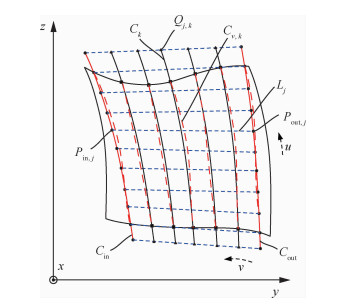图 4 曲面参数化过程 Fig. 4 Parametric process of surface

1) 分别在内外轮毂母线CinCout上等弧长提取相同个数的离散点Pin, jPout, j，并以点Pin, jPout, j为端点顺序连成线段Lj

2) 在全部的直线段Lj上等弧长提取相同个数的离散点Qj, k，并按列分别拟合成样条曲线Ck

3) 以曲线Ck为母线z轴为回转中心生成回转曲面。回转曲面族与设计曲面S(u, v)相交，交线为空间自由曲线Cv, k

4) 将曲线Cv, k按序沿参数u方向扫略生成曲面S(u, v)′。评价曲面S(u, v)′与原设计曲面之间的偏差，若偏差过大则需增加离散点Pin, jPout, jQj, k的数量重新构造曲面S(u, v)′。

1.3 砂布轮抛光路径规划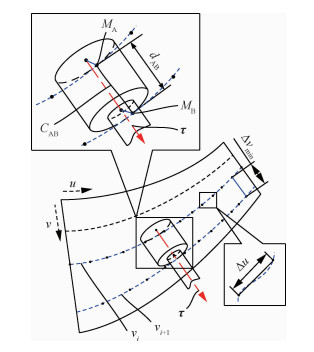图 5 曲面抛光路径规划 Fig. 5 Path planning of polishing surface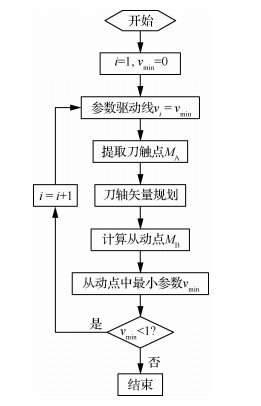图 6 曲面抛光路径规划流程 Fig. 6 Process of surface polishing path planning

2 刀轴矢量优化控制 2.1 压缩量偏差计算

 ${\delta _j} = {d_j} \times \sqrt {1 - {{\left( {{\mathit{\boldsymbol{n}}_j} - \mathit{\boldsymbol{\tau }}} \right)}^2}} - {R_{\rm{C}}}$ （1）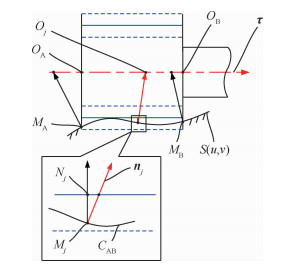图 7 接触曲线上压缩量偏差分布 Fig. 7 Distribution of compression deviation on contact curve

 $\mathit{\boldsymbol{n}}\left( {u,v} \right) = \pm \frac{{{\mathit{\boldsymbol{n}}_u}\left( {u,v} \right) \times {\mathit{\boldsymbol{n}}_v}\left( {u,v} \right)}}{{\left| {{\mathit{\boldsymbol{n}}_u}\left( {u,v} \right) \times {\mathit{\boldsymbol{n}}_v}\left( {u,v} \right)} \right|}}$ （2）

 $\left\{ \begin{array}{l} {\mathit{\boldsymbol{n}}_u}\left( {u,v} \right) = \frac{{\partial S\left( {u,v} \right)}}{{\partial u}}\\ {\mathit{\boldsymbol{n}}_v}\left( {u,v} \right) = \frac{{\partial S\left( {u,v} \right)}}{{\partial v}} \end{array} \right.$ （3）

2.2 最优刀轴矢量模型

 $\left\{ \begin{array}{l} {Q_{\rm{A}}} = {M_{\rm{A}}} + \left( {{R_{\rm{C}}} + a} \right) \cdot {\mathit{\boldsymbol{n}}_{\rm{A}}}\\ {Q_{\rm{B}}} = {{M'}_{\rm{B}}} + \left( {{R_{\rm{C}}} + b} \right) \cdot {{\mathit{\boldsymbol{n'}}}_{\rm{B}}} \end{array} \right.$ （4）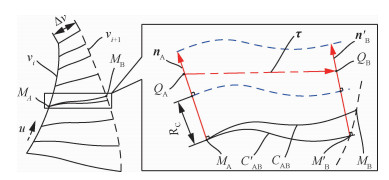图 8 刀轴方向参数化表示 Fig. 8 Tool orientation indicated by parameters

 $\mathit{\boldsymbol{t}} = \frac{{\overrightarrow {{Q_{\rm{A}}}{Q_{\rm{B}}}} }}{{\left| {\overrightarrow {{Q_{\rm{A}}}{Q_{\rm{B}}}} } \right|}}$ （5）

 $\left\{ \begin{array}{l} \mathit{\boldsymbol{\tau }} = \lambda \times \mathit{\boldsymbol{g}}\left( {a,b,c} \right)\\ \begin{array}{*{20}{c}} {\mathit{\boldsymbol{g}}\left( {a,b,c} \right) = \overrightarrow {{M_{\rm{A}}}{{M'}_{\rm{B}}}} + \left( {{R_{\rm{C}}} + b} \right) \cdot {{\mathit{\boldsymbol{n'}}}_{\rm{B}}} - }\\ {\left( {{R_{\rm{C}}} + a} \right) \cdot {\mathit{\boldsymbol{n}}_{\rm{A}}}} \end{array} \end{array} \right.$ （6）

 $\left\{ \begin{array}{l} {O_{\rm{A}}} = {M_{\rm{A}}} + \frac{{\left| {{M_{\rm{A}}}{Q_{\rm{A}}}} \right| \times \left( {{\mathit{\boldsymbol{n}}_{\rm{A}}} \cdot \mathit{\boldsymbol{\tau }}} \right) \cdot \mathit{\boldsymbol{\tau }}}}{{{{\left| \mathit{\boldsymbol{\tau }} \right|}^2}}}\\ {O_{\rm{B}}} = {O_{\rm{A}}} + {d_0}\tau \end{array} \right.$ （7）

 $\left\{ \begin{array}{l} {\delta _j} = \mu {f_j}\left( {a,b,c} \right) - {R_C}\\ {f_j}\left( {a,b,c} \right) = {d_j} \times \sqrt {1 - {{\left( {{\mathit{\boldsymbol{n}}_j} \cdot \frac{{{\mathit{\boldsymbol{g}}_j}\left( {a,b,c} \right)}}{{\left| {{\mathit{\boldsymbol{g}}_j}\left( {a,b,c} \right)} \right|}}} \right)}^2}} \end{array} \right.$ （8）

 $\min \left\{ {\max \left\{ {\left| {{\delta _j}} \right|} \right\}} \right\}$ （9）

 $\min f\left( {a,b,c} \right) = \max \left\{ {\left| {{f_j}\left( {a,b,c} \right)} \right|} \right\}$ （10）

2.3 刀轴矢量求解

 $\left\{ \begin{array}{l} v_{i,d}^k = wv_{i,d}^{k - 1} + {c_1}{r_1}\left( {{p_{i,d}} - x_{i,d}^{k - 1}} \right) + \\ \;\;\;\;\;\;\;\;\;\;{c_2}{r_2}\left( {{g_d} - x_{i,d}^{k - 1}} \right)\\ x_{i,d}^k = x_{i,d}^{k - 1} + v_{i,d}^{k - 1} \end{array} \right.$ （11）

1) 计算参数(a, b, c)的取值范围。参数ab代表压缩量偏差，则其应该满足0≤a≤[δmax]、0≤b≤[δmax]。而参数c为调整从动刀触点在参数u方向的位置，为避免相邻刀位点间刀轴矢量交叉，则其应该0≤c≤0.25Δu

2) 粒子群初始化。本文中的粒子属于低纬度，个数m可取值10 < m < 20。每一个粒子的参数初始值随机产生。而常数c1=c2=2，w=0.9，且在迭代时w按照10%的衰减率减小。最大速度一般限定为各分量取值范围的10%~20%。迭代终止条件为迭代次数达到最大值或者连续两次迭代的之间的差值小于给定的阈值。

3) 按照迭代公式进行迭代搜索，得到适应度最优的参数(a*, b*, c*)，并据此计算刀轴矢量和刀位点。

3 试验及结果分析 3.1 试验条件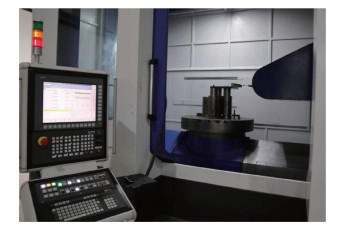图 9 试验验证 Fig. 9 Experimental verification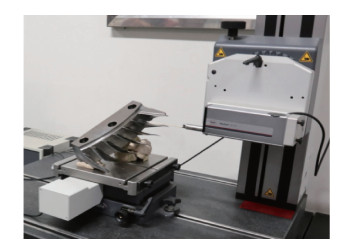图 10 表面轮廓检测 Fig. 10 Measurement of surface profile
3.2 试验结果

 抛光 RC/mm Δs/mm d0/mm [δmax] /mm 粗抛 14.60 0.8 10 0.05 精抛 14.96 0.6 10 0.05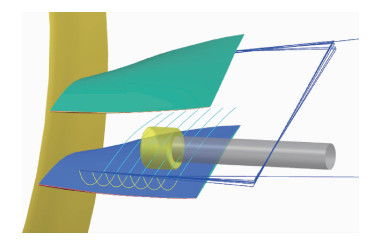图 11 抛光过程在UG中的仿真 Fig. 11 Simulation of polishing process in UG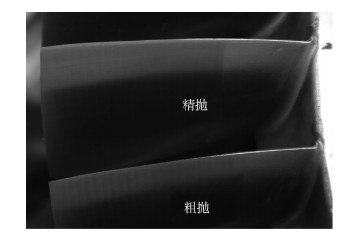图 12 曲面抛光效果 Fig. 12 Results of surface polishing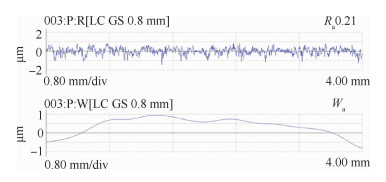图 13 表面轮廓检测结果 Fig. 13 Measurement results of surface profile

3.3 对比试验及分析

 方法 u=0.25 u=0.50 u=0.75 最大偏差/mm 平均偏差/mm 标准差/mm 最大偏差/mm 平均偏差/mm 标准差/mm 最大偏差/mm 平均偏差/mm 标准差/mm 文献 0.065 0.035 0.020 0.060 0.031 0.015 0.038 0.021 0.012 文献 0.055 0.034 0.015 0.051 0.025 0.012 0.039 0.015 0.012 本文 0.049 0.031 0.016 0.042 0.027 0.013 0.038 0.016 0.011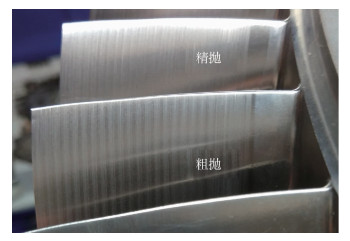图 14 文献中所提方法的抛光效果 Fig. 14 Polishing effect with the method in Ref.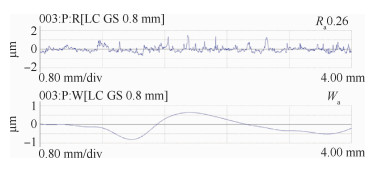图 15 文献中所提方法表面轮廓检测结果 Fig. 15 Measurement results of surface profile with the method in Ref.
4 结论

1) 建立了砂布轮与曲面挤压接触曲线上压缩量偏差分布模型。

2) 以压缩量偏差的最小化为目标建立刀轴矢量优化模型，并利用粒子群优化算法求解。

3) 基于最优刀轴矢量构建曲面上刀触轨迹曲线规划流程。

4) 检测结果表明，试件抛光区域的轮廓度、粗糙度均符合工艺要求。其中，粗糙度由0.26 μm降低至0.21 μm，并且表面轮廓曲线的一致性得到明显的改善。

http://dx.doi.org/10.7527/S1000-6893.2019.23330

0

#### 文章信息

WANG Zhiwei, LIN Xiaojun, SHI Yaoyao, GAO Yuan, ZHANG Yun

A polishing path planning method for blisk with abrasive cloth wheel based on compression deviation constraint

Acta Aeronautica et Astronautica Sinica, 2020, 41(2): 623330.
http://dx.doi.org/10.7527/S1000-6893.2019.23330